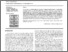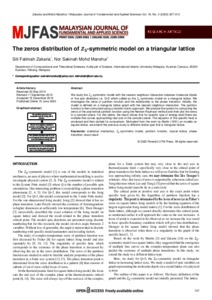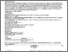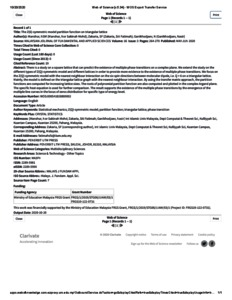# The zeros distribution of Z_5-symmetric model on a triangular lattice

Zakaria, Siti Fatimah and Mohd Manshur, Nor Sakinah (2020) The zeros distribution of Z_5-symmetric model on a triangular lattice. Malaysian Journal of Fundamental and Applied Sciences, 16(2020) (3). pp. 307-313. ISSN 2289 5981 E-ISSN 2289 599xPreview
PDF - Published VersionPreview
PDF (wos) - Supplemental Material

## Abstract

We study the -symmetric model with the nearest neighbour interaction between molecular dipole of five spin directions i.e. Q=5 which called as the -symmetric model on a triangular lattice. We investigate the zeros of partition function and the relationship to the phase transition. Initially, the model is defined on a triangular lattice graph with the nearest neighbour interaction. The partition function is then computed using a transfer matrix approach. We analyse the system by computing the zeros of the polynomial partition function using the Newton-Raphson method and then plot the zeros in a complex plane. For this lattice, the result shows that for specific type of energy level there are multiple line curves approaching real axis in the complex plane. The equation of the specific heat is produced and then plotted for comparison. Motivated from the work by Martin (1991) on models on square lattice, we extend the previous study to different lattice type that is triangular lattice.

Item Type: Article (Journal) 8033/80923 symmetric model ; Newton-Raphson ; Q Science > QA MathematicsQ Science > QC Physics Kulliyyah of Science > Department of Computational and Theoretical Sciences Dr Siti Fatimah Zakaria 22 Jun 2020 15:37 21 Jan 2021 09:07 http://irep.iium.edu.my/id/eprint/80923View Item